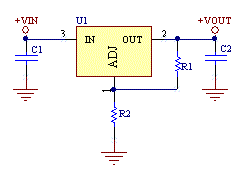# Adjustable Voltage Regulator Resistor Divider Calculator

This calculator computes the resistor divider network for most adjustable voltage regulators whether they are linear or switching.Voltage Regulator Circuit Schematic

 Result: Input: Reference Voltage (Vr) Output Voltage (V) R1 (KOhm) R2 (KOhm)

See our standard resistor calculator for a real world resistor value.

### Equations:

R2= (Vo-Vr)*R1/Vr

[Plant Database], [Soil Moisture Sensor] [Water Level Sensor] [Soil Moisture Meter]